Power BI Desktop gives you the right way to deal with your data in just a few clicks. However, the data does not contain everything you need to create reports. This is where measures come into play and will certainly help you to get most out of Power BI.

You can use measures in the most common data analysis, such as sums, averages, counts or in advanced calculations. With the help of Data Analysis Expressions (DAX) formula, you can create a measure having calculated in response to your data and interaction with your reports. This article is for Power BI users already familiar with using Power BI Desktop to create more advanced models.

Insight on measures
The Power BI desktop allows you to create and use measures in Report View or Data View. The created measures appear in the fields list with a calculator icon. Measures can be named with any required name based on your choice, and further you can use visualization for a better look and feel.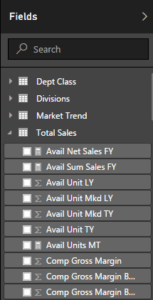Measures calculate a result using DAX formula, which includes various set of functions, operator, and constructs giving elasticity in creating measures to calculate results for data need. DAX formulas are bit similar to Excel formulas and have many of the same functions like DATE, SUM, and LEFT. However, DAX’s functions are calculated with relational data like in Power BI Desktop.

Example: Create your own measure to calculate variance

Let’s try out an example of creating a measure to calculate variance of two columns from a table “Total Sales”. One column represents the sum of total amount for this year and other the sum of total amount for last year, so now we will create two measures having sum of both columns and the third measure will represent the variance of last year vs this year.

1. Start with creating a new measure by clicking on the New Measure button in the ribbon on Power BI Desktop’s Home tab.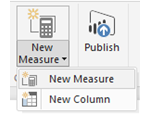Once you click, a formula bar will appear on the top, this is where you can rename and enter a DAX formula for our measure.2. As in this case, you are calculating the Total Sales Revenue for This Year and Last Year. So, first create a measure to calculate the Total Sales for This Year by dividing the Sum of Sales Amount TY by Sum of Sales Units TY. This DAX equation will give the total sales for TY.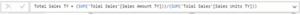3. After calculating the result, represent the values using Bar Chart visualization. You can simply drag the Total Sales TY field from Total Sales table to chart values.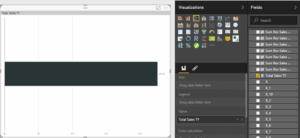You can easily visualize the calculated data of a created measure.

4. Now create a second measure calculating the values for Total Sales Last Year. Similarly, divide the sum of Sales Amount LY with a sum of Sales Units LY to get the values for Total Sales LY.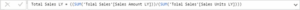5. Now to show the slight comparison in the bar chart, you can simply drag the Total Sales LY filled from Total Sales table to the same chart under values section.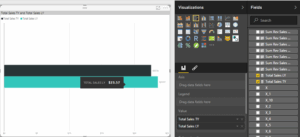You can visualize the comparison between total sales last year and this year in chosen bar chart.

6. Finally, to calculate the variance of above calculated fields, create a new measure named as VAR Total % or you can rename the measure accordingly. This measure will calculate the variance percentage from the created measure fields Total Sales TY and Total Sales LY.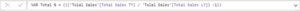7. You can highlight the calculated values using Card item from Visualization. Simply drag the VAR Total % under visual level filters. The card will represent the Variance of Total Sales This Year Vs Last Year.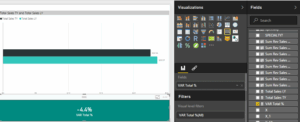You can play with visualization of a card using format pencil icon and change the color combination, length, height, background, etc.

Few important tips on measures:

1. To create a new measure under the same table, you can either select New Measure from the ribbon or right-click on table in the field list, and then click New Measure. This will create your new measure under the same table, where it will be easier to find.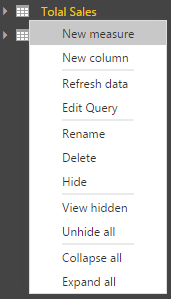2. In Power BI desktop, if you are dividing two columns and one of the columns is having a zero value, then you can rectify the issue using IFERROR statement having following DAX function.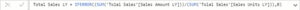This expression will give you an error in case of having zero value in either of the columns.
Note: You cannot divide the columns containing zero values.

3. You should use appropriate parenthesis while creating a measure. In most cases, users will face Syntax error due to missing or misplaced closing parenthesis.

This blog emphasizes the power of measures giving the results desired from the data. As illustrated, you can name measures as it makes sense to you, and you can easily use the table suggestion list to find and select the right element to add in our formulas. Further, you can use them in your report canvas with better visualization and results.